# Ranch House Basic Takeoff Walkthrough

A basic takeoff refers to measurements for different areas of a construction blueprint plan. These measurements will be “taken off” the blueprint using computer programs such as Bluebeam or On Screen Takeoff. With these rough dimensions, you can start to estimate quantities of material and labor required to complete the project.

Hint: You may want to use the Spreadsheet Calculator you created previously to help with these calculations.

## Assignment Overview

### Scope of Work

Use Bluebeam to take measurements and calculate estimates for a construction project from the project plans. The required estimates you will provide should include the following:

#### BasicInfo Tab

• Main Floor Area
• Basement Floor Area
• Garage Floor Area
• Exterior Wall Footing Length
• Garage\Patio Wall Footing Length
• Basement Foundation Length
• Shallow Foundation Length
• 2x4 Basement Bearing Wall Length
• 2x4 Basement Insulation Wall Length
• 2x6 Exterior Wall Length
• 2x4 Garage Wall Length
• 2x6 Common Wall Length
• 2x4 Interior Wall Length
• 2x6 Interior Wall Length
• Flat Roof Area
• Lineal Feet Roof Edge
• Lineal Feet Roof Ridge Line

## Determine the Sequence and Units of Measure for the Basic Takeoff

The first step is to decide the order in which you will measure elements of the plans, and the units you will use to measure them.

### Step 01: Determine the Sequence for Completing the Basic Takeoff

Use the following sequence for basic takeoffs:

1. Square Footage of Floor Area
2. Square Footage of Flat Area Covered by Roof
3. Lineal feet of Footings
4. Lineal Feet of Foundation
5. Lineal Feet of Interior Wall
6. Lineal Feet of Exterior Wall
7. Lineal Feet of Common Wall
8. Lineal Feet of Garage Wall
9. Lineal Feet of Roof Edge
10. Lineal Feet of Roof Ridge Line
11. Door Schedule
12. Window Schedule

### Step 02: Identify the Units of Measure for Structure Components

The units of measurement for the basic takeoff are found in the sequence above. It is vital to recognize these units because they will be used to estimate both material quantity and labor.

## Estimate the Square Footage of the Floor Area from the Plans

### Step 01: Measure the Main Floor Living Area

Calculate the space based on the exterior wall dimensions, not interior wall dimensions.

• If the exterior walls of the main floor area do not form a simple rectangle, break the main floor area into simple geometric shapes which can then be measured in square feet and added together to get a total square footage.

#### Example

• Figure 5-27 Shows a graphic of the first floor with the main floor area highlighted in light tan and the garage area in gray.
• Notice that Figure 5-27’s dimensions from the takeoff in Bluebeam use the outside of the exterior walls.
• Because the main floor area is a simple rectangle, the square footage will be calculated using a single equation. 36’ x 32’ = 1,152 ft2.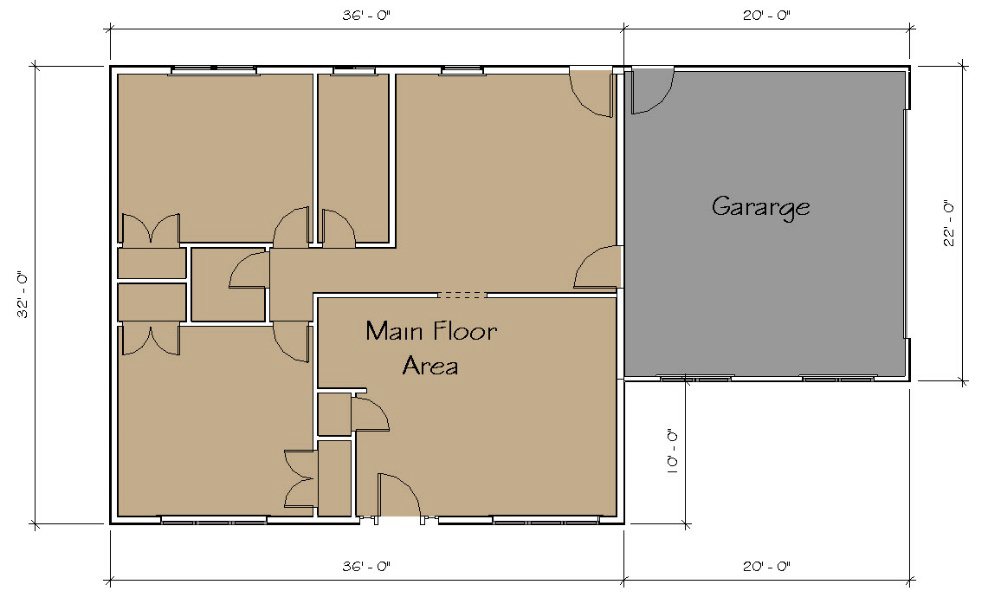Figure 5-27 Graphic representation of the main floor and garage area of the building.

### Step 02: Measure the Garage Floor Area

1. Calculate the space based on the exterior wall dimensions, not interior wall dimensions.
• This process is basically the same as that for calculating the main floor area.
• Based on the takeoffs in Figure 5-27 above, the area of the garage flooring is 440 ft2.

### Step 03: Measure the Basement Floor Area

Calculate the basement based on the INTERIOR wall dimensions.

• This calculation includes the area of any storage area under a porch and any doorways formed in the concrete of the basement.

#### Example

• Figure 5-28 Shows a graphic of the basement plans for a structure.
• Notice that this figure’s dimensions from the takeoff in Bluebeam use the inside of the basement walls.
• This figure shows a basement area, a porch area, and dimensions for the doorway.
• The formula for calculating the total area of this figure is as follows:
• Basement Area + Porch Area + Doorway Area = Total Area
• (34’-8” x 30’-8”) + (9’-8” x 5’-7”) + (3’-2” x 8”) = Total Area
• 1063.11 ft2 + 53.97 ft2 + 2.11 ft2 = 1119.19 ft2
• The total area of the basement is 1119.19 ft2.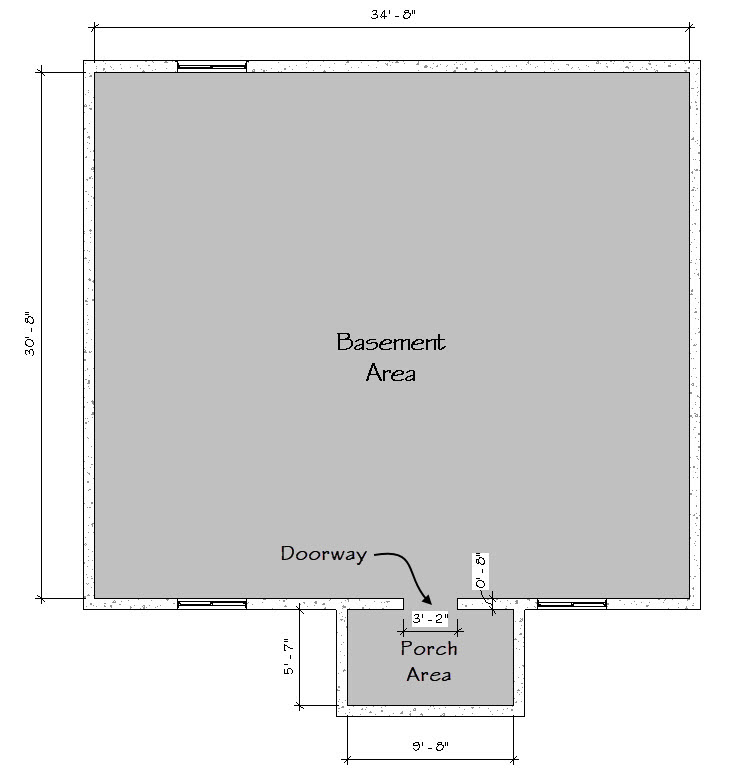Figure 5-28 The basement floor area square footage is comprised of three separate floor areas.

### Step 04: Enter the Floor Area into the Estimating Worksheet

Take the numbers you calculated for the various floor areas of the plans and enter them in the appropriate cells of the BasicInfo tab in the Estimating Worksheet.

#### Example

The numbers calculated from the graphics in Figure 5-27 and Figure 5-28 will be entered in the BasicInfo tab, as shown in Figure 5-30.

## Estimate the Lineal Feet of the Footing from the Plans

The next basic takeoff to be completed will be the footing section. There are two distinct styles of footing; exterior wall footings and the interior grade footing.

### Step 01: Measure the Exterior Wall Footings

Calculate the length of the exterior wall footings. This is more complicated than it seems.

• Exterior wall footings are usually built to a specific standard.
• For example, residential buildings likely have standardized exterior footings that are16” wide and 8” deep (Figure 5-33).
• Because these footings have the added dimension of width, they must be calculated to account for overlap between any corners. Failing to do so will result in inaccurate footing estimates because the square area of each corner will have the lineal feet measurement counted twice.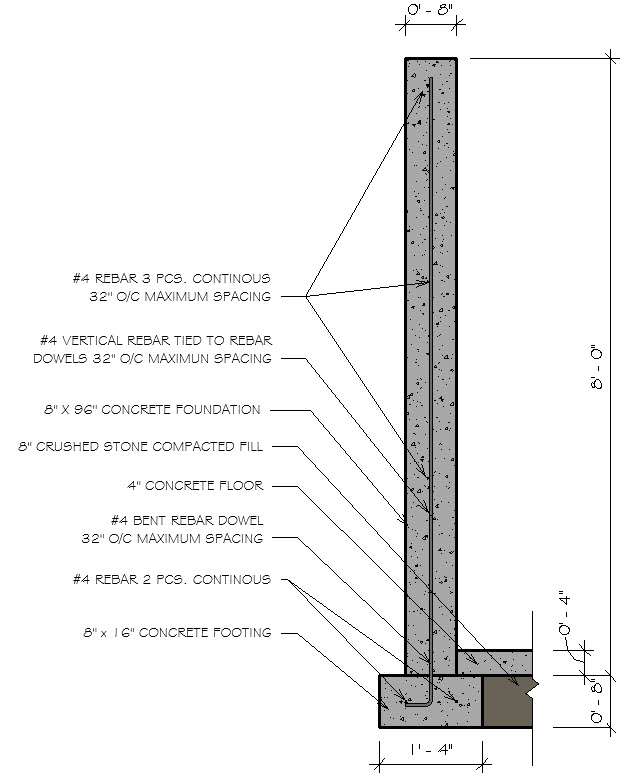Figure 5-33 Footing and foundation wall section detailing the construction specifics.

#### Methods for Calculating Footings

This guide will offer two different methods for calculating the length of concrete footings. Examples for each method will be demonstrated using Figure 5-34.

#### Method 01: Averaging the exterior and interior dimensions.

1. Measure both the exterior dimensions and interior dimensions of the footings.
2. Add both dimensions together and divide in half to find the average length. This is the estimated length of the footing.

(Exterior Dimension + Interior Dimension) ÷ 2 = Average Lineal Feet

Examples

• The leftmost footing in Figure 5-34 has an exterior length of 32’ and an interior length of 30’.

(Exterior Dimension + Interior Dimension) ÷ 2 = Average Lineal Feet

(32’ + 30’) ÷ 2 = Average Lineal Feet

62’ ÷ 2 = 31 Lineal Feet

• The topmost footing in Figure 5-34 has an exterior length of 36’8” and an interior length of 34’.

(36’-8” + 34’) ÷ 2 = Average Lineal Feet

70’-8” ÷ 2 = 35’-4” Lineal Feet

• The total lineal feet for these two footings would be 66’-4” using this method.

#### Method 02: Break the footings into individual pieces using Bluebeam and measure their length.

1. Break the footings into individual pieces that are placed on the page horizontally and vertically.
2. Calculate the horizontal footing by measuring to the outside of the footing the vertical footings by measuring to the inside of the footings.

Examples

• Notice that Figure 5-34 shows a dimensioned plan view of the footings.
• The horizontal footings on the page are dimensioned to the outside of the wall and are highlighted in yellow.
• The vertical footings on the page are dimensioned to the inside of the wall and are highlighted in green.
• The horizontal garage footings are measured to the basement foundation.

The leftmost vertical footing has a length of 30’ highlighted in green.

The topmost horizontal footing has a length of 36’-8” highlighted in yellow.

• The total lineal feet for these two footings would be 66’-8”.

Notice how the total lineal feet for these two footings are slightly different between the two methods. In general, using Method 01 might be easier if you do not have the ability to highlight and measure areas in the plans. Method 02 is a bit more accurate and should be used if possible.

Total Calculations for Figure 5-34: Using Option 02 and the Spreadsheet Calculator you made previously, the total lineal feet of the exterior footings is 215.50 LF, as illustrated in the graphic below.

### Step 02: Measure the Interior Wall Footings

Calculate the length of the interior wall footings.

• Use the same method used to calculate the exterior wall footings.
• Interior and exterior wall footings are separated in a basic takeoff because they may have the same dimensions, but different requirements for reinforcement with rebar. Because of this, the cost of materials and labor per lineal foot is different for each type of footing.
• Figure 5-32 shows the difference in reinforcement for interior versus exterior wall footings.

### Step 03: Enter the Total Exterior and Interior Wall Footing Lengths

The total lengths of exterior and interior wall footings should be entered in the respective cells of the BasicInfo tab of the Estimating Worksheet.

## Estimate the Lineal Feet of the Foundation from the Plans

The next basic takeoff to be completed will be the foundation section. There are two distinct styles of foundation; main foundation and the garage foundation. Each of these styles have different requirements for dimensions, as illustrated in Figure 5-31.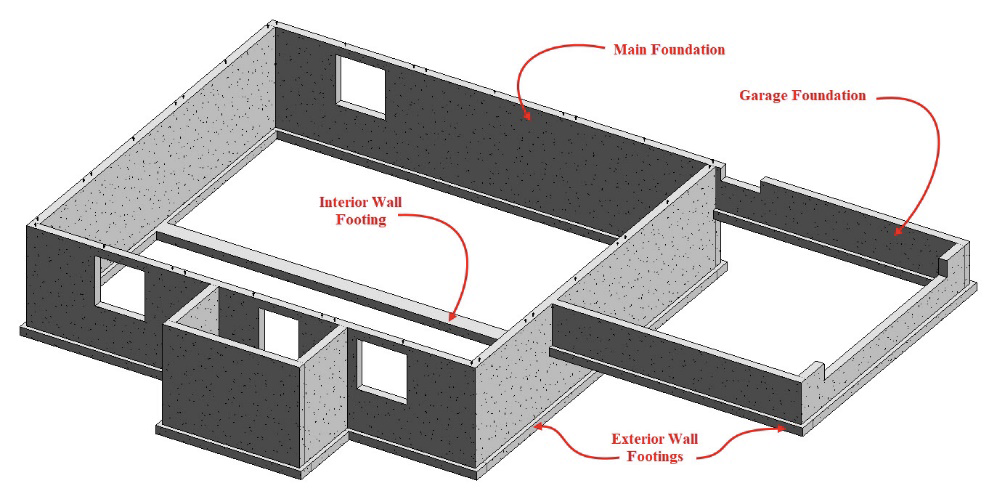Figure 5-31 Three dimension footing and foundation view showing different footing and foundation styles.

### Step 01: Measure the Main Foundation

Calculate the length of the main foundation.

• This process carries the same complications as calculating exterior footings.
• Use Method 02 from the Strategies for Calculating Footings section above.

Example

• Figure 5-36 is a graphic of the foundation from the same structure used as an example for calculating footings.
• The foundations which are placed horizontally on the page are dimensioned to the outside of the walls and are highlighted in dark blue.
• The foundations placed vertically on the page are dimensioned to the inside of the wall and are highlighted in light blue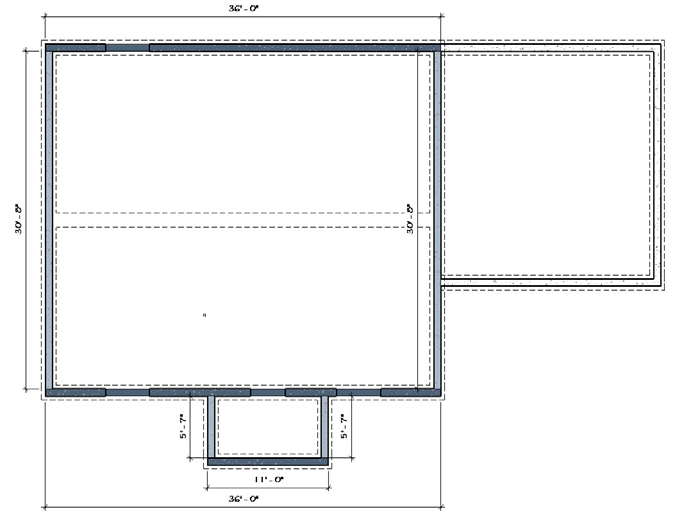Figure 5-36 Graphic showing the lineal footage of foundation floor plan.
• Using the lengths provided by the highlights, the following numbers can be added up to calculate a total of 155.50 LF of Main Foundation.

### Step 02: Measure the Garage Foundation

Calculate the length of the garage foundation.

• This process is similar to that used to calculate the main foundation length.
• Because the two different types of foundation have different dimension requirements, they must be calculated separately. Failure to do so will result in inaccurate estimates for material and labor.

### Step 03: Enter the Total Main and Garage Foundation Lengths

The total lengths of main and garage foundations should be entered in the respective cells of the BasicInfo tab of the Estimating Worksheet.

## Estimate the Lineal Feet of the Various Walls from the Plans

The wall takeoffs will be completed in similar fashion as the footing and foundation takeoffs. There are a number of different wall type. There are also a number of different ways of classifying the walls. For purposes of this assignment, walls will be classified into three basic categories. These categories are Basement Walls, Exterior Walls, and Interior Walls. Each of these categories will include different kinds of walls  that are estimated separately because they vary in the specifications and materials used to construct them.

### Step 01: Identify the Different Wall Types Used in the Basement

Look at the construction plans and identify the types of walls used in the basement. Some criteria for identifying wall types includes:

• Is the wall load bearing, meaning it supports the structure above it, such as a floor or roof? These types of walls have a double top plate and are framed using 2x4’s spaced 16 inches on center.
• Is the wall framed to provide a place to install insulation? These types of walls are framed using a single top plate with a stud spacing of 24 inches on center.

There are two types of basement walls that must be measured and estimated separately. They include Basement Bearing Walls and Basement Insulation Walls.

#### Basement Bearing Walls

• These are framed in the basement or on the foundation to support the floor system.
• Walls to frame out the various basement rooms are included in this category.
• Pony walls (shorter walls that sit on top of the foundation to complete the basement wall structure) are also included in this category.

#### Basement Insultation Walls

• These are walls framed around the inside of the foundation to provide a structure for installing insulation and wall finishes.

Figure 5-39 Shows the basement walls of the project used as an example in this guide.

The actual construction of each wall type can best be determined by referencing building sections or wall section detail drawings. Most often notes and annotations will detail the wall construction similar to what was previously shown when calculating the footing and foundation walls. Figure 5-40 Shows and expanded section view of the footing and foundation wall section shown in Figure 5-33. The view shows the addition of the basement insulation wall.

### Step 02: Measure the Height and Length of the Various Basement Walls

Calculate the height and length of the Basement Walls.

• This process carries the same complications as calculating exterior footings.
• Use Method 02 from the Strategies for Calculating Footings section above.

Example

• Figure 5-41 is a graphic of the basement walls from the same structure used as an example for calculating footings and foundations.
• The Insulation Walls are highlighted in pink, and the Bearing Walls are highlighted in blue.
• Notice that the various walls include blocks so that the corners where two walls join are not counted twice.
• Using the lengths provided by the highlights of the Bearing Walls, the following numbers can be added up to calculate a total of 63.75 LF of Basement Bearing Walls.

### Step 03: Identify the Different Wall Types Used in the Exterior Structure

There are three types of walls in the Exterior Walls category that must be estimated separately.

#### 2x6 Exterior Walls

• These walls are built on the floor structure and could be framed as a single wall.
• These walls are sheathed with OSB sheathing.

#### 2x4 Garage Walls

•  These walls are built on the garage foundation.
• These walls are built using 2x4’s as opposed to 2x6’s.
• The studs are longer than other exterior wall types because they go all of the way to the foundation instead of resting on the floor system.
• The bottom plates of these walls are pressure treated material.

#### 2x6 Common Walls

• These walls are built on the floor structure and could be framed as a single wall.
• These walls are covered with dry wall on both sides.
• These walls act as a shared barrier between 2x6 Exterior Walls and 2x4 Garage Walls
• Because of the difference in materials used (OSB versus drywall) Common Walls are estimated separately from Exterior Walls.Figure 5-43 Exterior corner showing the intersection of three exterior wall types.

### Step 04: Measure the Height and Length of the Various Exterior Walls

The heights and total lengths of the exterior walls should be entered in the respective cells of the BasicInfo tab of the Estimating Worksheet.

### Step 05: Identify the Different Wall Types in the Interior of the Structure

There are three types of walls in the Interior Walls category that must be estimated separately.

#### 2x4 Interior Walls

• These walls are found inside the exterior walls and are built on the floor structure of 2x4 studs.

#### 2x6 Interior Walls

•  These walls are found inside the exterior walls and are built on the floor structure of 2x6 studs.

#### 2x4 Tall Interior Walls

• These walls are found inside the exterior walls and are built on the floor structure of 2x4 studs.
• These walls are taller than the height of the exterior walls and are typically found with vaulted ceilings.

### Step 06: Measure the Height and Length of the Various Interior Walls

The total lengths of main and garage foundations should be entered in the respective cells of the BasicInfo tab of the Estimating Worksheet.

### Step 07: Enter the Measurements of the Various Walls into the Worksheet

The total lengths and heights of 2x6 Exterior Walls, 2x4 Garage Walls, and 2x6 Common Walls should be entered in the respective cells of the BasicInfo tab of the Estimating Worksheet.

## Complete the Roof Takeoff

The roof subsection of the basic takeoffs is comprised of three quantities which are, square footage of flat roof area, lineal feet of roof ridge, and lineal feet of roof edge.

### Step 01: Identify and Measure the Flat Roof Dimensions

Identify the flat roof dimensions.

• The footprint of the roof is used to calculate the roof dimensions, not the actual area of each sloped side of the roof.
• This number includes all of the roof area, including overhangs.
• Be careful to identify any sections of roof that are covered by the overhangs of other roof sections. They still must be accounted for when calculating the total flat roof area.

Example

• Figure 5-46 Shows a dimensioned roof plan.
• Notice that the roof plan does not show a small area of the garage roof that is under the main roof. This roof area is equal to the distance of the roof overhang multiplied by half of the roof width. This is highlighted by the area outlined in red.

### Step 02: Segment the Flat Roof Area and Calculate the Dimensions

Figure 5-46 above is already segmented into multiple areas that can be entered into the Spreadsheet Calculator and added up to find the total flat roof area. (Figure 5-47)

The total flat roof area of the structure in Figure 5-46 is 1,779.45 SF.

### Step 03: Estimate the Lineal Footage of the Roof Ridge Line

The roof plan in Figure 5-46 Can also be used to determine the lineal footage of roof ridge line.

• Three ridge lines are shown: the main roof ridgeline, the garage roof ridgeline, and the front porch ridgeline.
• The length of both the main roof and garage ridge lines can be determined from the dimensions.
• To determine the length of the front porch ridgeline, add the 5’-10 ¼” roof edge distance to the roof run to find the total length.
• In this case, the span of the roof is 12’-1 ½” and the run would be half of that distance, or 6’-0 ¾”. The total length of the front porch ridgeline would be:

5’-10 ¼” + 6’- 0 ¾” = 11’- 11”

Figure 5-48 Shows the lineal footage of roof ridge line entered into the spreadsheet calculator.

### Step 04: Estimate the Lineal Footage of the Roof Edge

The lineal footage of roof edge is a little more complicated than the other two roof basic takeoffs.

• This takeoff follows the edge of the roof around the building, and includes both the horizontal roof edges and sloped roof edges.

Example

Figure 5-49 Shows the East Side elevation of the example structure used in this walkthrough and highlights the roof edges in red.

#### Calculate Horizontal Roof Edges

• The single horizontal roof can be determined from the 6’-3’ dimension.

#### Calculate the Sloped Roof Edges

First, identify the Roof Slope.

• The roof slope in Figure 5-49 is shown as 6:12.
• The slope length of the roof is a triangle that is half of the roof span.

Next, identify and measure the each Roof Span.

• The smaller garage span is measured at 22'-0".
• The larger house span is determined by calculating the garage and the addition width of the house. This gives the roof ridge of the house a span of 32'-0".

Next, calculate the Run of each roof edge.

• The Run of a roof is the length of a horizontal line that would form a right triangle with a vertical line from the highest point of a roof edge and the roof slope.
• An easy way to calculate the Run of a roof is to divided the Span in half.
• The run of the smaller garage Span in Figure 5-49 can be calculated as follows:

22’- 0” ÷ 2 = 11’-0” Run

• The larger house Span is determined by calculating the garage and the additional width of the house. This gives the roof ridge of the house a span of 32'-0". The run can be calculated as follows:

32’- 0” ÷ 2 = 16’- 0” Run

Next, calculate the Rise of each roof edge.

• The run and the roof slope ratio can be used to calculate the vertical roof rise.
• Multiply the run by the slope ratio, which is given in Figure 5-49.
• The garage roof rise can be calculated by the following:

6/12 Ratio x 11’-0” Run

Or

½ x 11’- 0” = 5’- 6” Rise

• The main house roof rise can be calculated by the following:

6/12 Ratio x 16’- 0” Run

or

½ x 16’- 0” – 8’- 0” Rise

The run and rise of each section can be put into the spreadsheet calculator and used to calculate the slope length. This is shown in Figure 5-50.

Calculate the total roof edge using the Run and Rise dimensions.

• Entering the Run and Rise dimensions into the spreadsheet calculator should calculate the Hypotenuse of each triangle.
• These Hypotenuse lenths will be the roof edge lengths.

Example

• The total roof edge on the side of the house shown in Figure 5-49 would be equal to the sloped roof edges of the house multiplied by two, and one garage sloped roof edge.
• Also add the horizontal run of the side of the front porch.
• The three sloped lengths are added the horizontal length to calculated the total length of the roof edge on this side of the house. The total would be as follows:

17.89’ + 17.89’ + 12.3’ + 6.25’ = 54.33’

• This total would be added to the totals for the other side of the roof to find the total length of roof edge. In the case of the example, this would be 186.00 LF.

### Step 05: Enter the Roof Takeoffs into the Worksheet

The square footage of the flat roof area and the total lineal footage of the roof ridge line and roof edge should be entered in the respective cells of the BasicInfo tab of the Estimating Worksheet.

For example, the inputs for the Roofs basic takeoff for the example structure is shown in Figure 5-52.

## Complete the Window and Door Basic Takeoffs

The majority of information to complete both the window and door takeoffs can be obtained from the door and window schedules.

### Step 01: Copy the Information from the Door and Window Schedules into the BasicInfo Tab

Copy the information into the appropriate section in the Basic takeoffs.

• Figures 5-53  and 5-55 show the window schedule and the door schedule, respectively, for the structure used as an example throughout this walkthrough.
• The information entered into the basic takeoffs is shown in Figures 5-54 and 5-56.

## Check Your Work and Submit the Basic Takeoff

• Save your Estimating Template with the Basic Info worksheets completed for the assigned project plans.
• Name your Estimating Template with your first and last name in the title followed by “Basic Takeoff Assignment”

Example: John Doe Basic Takeoff Assignment

• Submit your completed Estimating Template.